Two-dimensional modelsNext: Conclusions Up: No Title Previous: Dynamic Model

# Two-dimensional models

In previous sections, we studied the traffic flow in one dimension. However, in cities the problem is much more complex. One has to consider the effect of intersection, traffic lights, coupling of traffic in different directions, turning cars, etc. Several two-dimensional models were advanced, which allows to incorporate those effects to a different extent.
A simple CA model in two dimensions was developed by Biham el al. . The model is defined on a square lattice with periodic boundary conditions. Each site can be in three states, it contains a car moving upwards, a car moving to the right or it is empty. The dynamics is controlled by traffic lights so that cars can move to the right at odd time steps and upwards on the even time steps. In one time step, cars can only move to the next site if this is empty.
The described system exhibits two different regimes. At low densities, one finds a dynamic phase, where the system self-organizes similar cars along the diagonal and therefore maximizes the average speed (see Fig. 7). At high densities, all cars are jammed in one big cluster, which leads to an average velocity of zero. The two phases are separated by a sharp transition at a critical density, which is size dependent.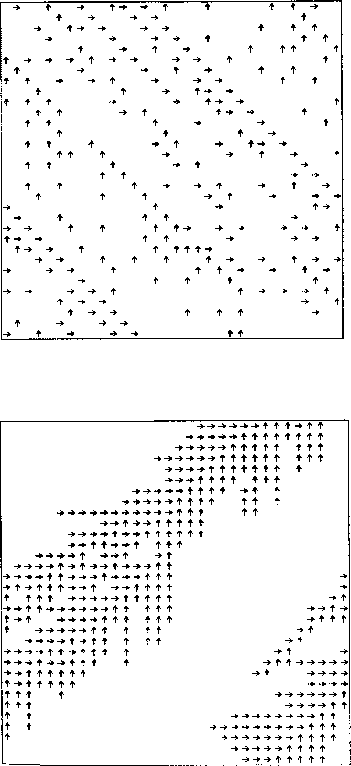Figure 7:  Two configurations of in Bihams two-dimensional cellular automata model by Biham el al. , a jam-free phase in the upper figure and congested phases below. The arrows denote the direction of moving cars.

In the model described above, cars can never turn. This possibility was included in a more advanced model by Cuesta el al. [13, 14]. There, a parameter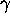is introduced, which determines the direction cars try to move. For one half of the cars,specifies the probability of trying to move upwards and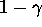the probability of trying to move to the right. For the other half of the cars, the opposite probabilities are assigned. Setting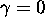, this reproduces Biham's model. An increasedgives car the possibility to take advantage of open sites out of their main direction of motion. This flexibility shifts the jamming transition to a higher density. This is shown in Fig. 8, which can be explained in terms of a first order transition critical point between.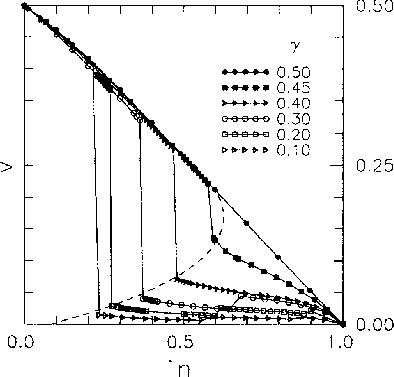Figure 8:  Average velocity versus car density at different randomness valueby Cuesta el al. 

In a following paper , the same system was studied using a non-linear Boltzmann equation. This approach can predict the existence of the jamming transition and physical quantities but does not show the correctdependence.

A much more elaborate model with realistic traffic rules was given by Freund el al. . In this model, a city is represented by L vertical and horizontal two-way streets, which leads to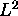crossing. At each crossing, cars can line up forming queues in every direction with a maximum length Q. There are no traffic lights but cars follow the left-before-right traffic rule, which is standard in many countries (for details see ). Each time a car lines up at a crossing the desired direction is chosen at random. A car can only cross an intersection if it has the right of way and the desired street is not fully occupied.

Numerical studies in  show a sharp jamming transition near a critical car density of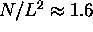(Fig. 9). Above this density, one still finds a remanent average velocity, which indicates that some jams may dissolve under those rules.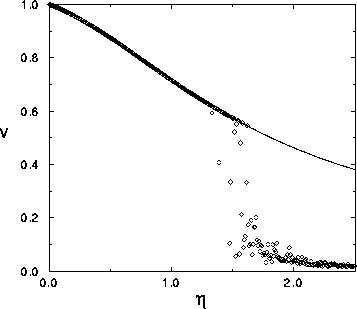Figure 9:  Average velocity versus density from simulation (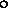) and statistical methods (solid line) by Freund el al. 

The numerical results were compared to predictions from statistical theory, in which the two-dimensional flow was described by a Bernoulli-process. The agreement is remarkably good until the jamming transition occurs (see Fig. 9).Next: Conclusions Up: No Title Previous: Dynamic Model

Burkhard Militzer
Sat May 9 11:34:31 CDT 1998